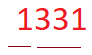# Cubes and cube roots class 8 ncert solutions Exercise 7.2

In this page we have Cubes and cube roots class 8 ncert solutions Exercise 7.2 for EXERCISE 7.2. This exercise has questions about cube root of the nunber, How to find it using Prime factorization and estimation method, True and False about properties of Cube Roots.Hope you like them and do not forget to like , social share and comment at the end of the page.

## Cubes and cube roots class 8 ncert solutions Ex 7.2

Question 1
Find the cube root of each of the following numbers by prime factorization method.
(i) 64
(ii) 512
(iii) 10648
(iv) 27000
(v) 15625
(vi) 13824
(vii) 110592
(viii) 46656
(ix) 175616
(x) 91125
Converting the number into common factors
(i)  64 = 2 x 2 x 2 x 2 x 2 x 2
= 23 x 23
3√64 =   2×2=4
(ii) 512 = 2 x 2 x 2 x 2 x 2 x 2 x 2 x 2 x 2
= 23 x 23 x 23
3√512 =   2×2×2=8
(iii) 10648 = 2 x 2 x 2 x 11 x 11 x 11
= 23 x 113
3√10648 =   2×11=22
(iv)  27000 = 2 x 2 x 2 x 3 x 3 x 3 x 5 x 5 x 5
= 23 x 33 x 53
3√27000 =   2×3×5=30
(v) 15625 = 5 x 5 x 5 x 5 x 5 x 5
= 53 x 53
3√15625 =   5×5=25
(vi) 13824 = 2 x 2 x 2 x 2 x 2 x 2 x 2 x 2 x 2 x 3 x 3 x 3
= 23 x 23 x 23 x 33
3√13824 =   2×2×2×3=24
(vii) 110592 = 23 x 23 x 23 x 23 x 33
3√110592 =   2×2×2×2×3=48
(viii) 46656 = 2 x 2 x 2 x 2 x 2 x 2 x 3 x 3 x 3 x 3 x 3 x 3
= 23 x 23 x 33 x 33
3√46656 =   2×2×3×3=36
(ix) 175616 = 23 x 23 x 23 x 73
3√175616 =   2×2×2×7=56
(x) 91125 = 53 x 33 x 33
3√91125 =   5×3×3=45

Question 2
State true or false.
(i) Cube of any odd number is even.
(ii) A perfect cube does not end with two zeros.
(iii) If square of a number ends with 5, then its cube ends with 25.
(iv) There is no perfect cube which ends with 8.
(v) The cube of a two digit number may be a three digit number.
(vi) The cube of a two digit number may have seven or more digits.
(vii) The cube of a single digit number may be a single digit number.

(i)As Odd multiplied by odd is always odd,So this statement is false
(ii)A perfect cube will end with odd number of zeroes for example 10,the cube will be 1000.So this statement is true
(iii) False
(iv) As 2= 8, This statement is false
(v) The smallest two digit number is 10 and 10= 1000 is a not three digit number.So this statement is false
(vi) 99 is the largest 2 digit number; 99= 989901 is a 6 digit number.So this statement is false
(vii)As 2= 8 is a single digit number, This statement is true

Question 3
You are told that 1,331 is a perfect cube. Can you guess without factorization what is its cube root? Similarly, guess the cube roots of 4913, 12167, 32768
We can estimate the cube root by the splitting the number from the right into three digit numbersSo for 1331
Left group 1
Right group 331
As you know 1= 1 so there would be 1 at unit’s place in cube root of 1331.
Now we have to find the cube root of left group 1
Now 1= 1
So, we have 1 in ten’s place and 1 in unit place
11= 1331 satisfies the condition
Cube Root of 4913
Right group = 913
Left group = 4
73 gives 3 at unit’s place so unit digit number in cube root of 4913 should be 7
We have to estimate the cube root of left group i.e 4
1= 1 and 2= 8
1<4<8
So, 10s digit in cube root of 4913 should be 1

## Summary

1. Cubes and cube roots class 8 ncert solutions Exercise 7.2 has been prepared by Expert with utmost care. If you find any mistake.Please do provide feedback on mail. You can download the solutions as PDF in the below Link also
2. This chapter 7 has total 2 Exercise 7.1 and 7.2. This is the last exercise in the chapter.You can explore previous exercise of this chapter by clicking the link below